https://doi.org/10.5194/essd-14-2767-2022
https://doi.org/10.5194/essd-14-2767-2022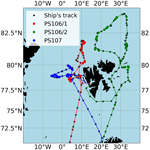# A dataset of microphysical cloud parameters, retrieved from Fourier-transform infrared (FTIR) emission spectra measured in Arctic summer 2017

Philipp Richter, Mathias Palm, Christine Weinzierl, Hannes Griesche, Penny M. Rowe, and Justus Notholt
Abstract

A dataset of microphysical cloud parameters from optically thin clouds, retrieved from infrared spectral radiances measured in summer 2017 in the Arctic, is presented. Measurements were performed using a mobile Fourier-transform infrared (FTIR) spectrometer which was carried by RV Polarstern. The dataset contains retrieved optical depths and effective radii of ice and liquid water, from which the liquid water path and ice water path are calculated. The water paths and the effective radii retrieved from the FTIR measurements are compared with derived quantities from a combined cloud radar, lidar and microwave radiometer measurement synergy retrieval, called Cloudnet. The purpose of this comparison is to benchmark the infrared retrieval data against the established Cloudnet retrieval. For the liquid water path, the data correlate, showing a mean bias of 2.48 g m−2 and a root-mean-square error of 10.43 g m−2. It follows that the infrared retrieval is able to determine the liquid water path. Although liquid water path retrievals from the Cloudnet retrieval data come with an uncertainty of at least 20 g m−2, a root-mean-square error of 9.48 g m−2 for clouds with a liquid water path of at most 20 g m−2 is found. This indicates that the liquid water paths, especially of thin clouds, of the Cloudnet retrieval can be determined with higher accuracy than expected. Apart from this, the dataset of microphysical cloud properties presented here allows researchers to perform calculations of the cloud radiative effects when the Cloudnet data from the campaign are not available, which was the case from 22 July 2017 until 19 August 2017. The dataset is published at PANGAEA .

Share
Dates
1 Introduction

Clouds play an important role in the radiation budget of the earth. In the visible regime, clouds mainly reflect and prevent solar radiation from reaching earth's surface, whereas in the thermal regime clouds prevent surface radiation from escaping to space and re-emit it back to earth, where it warms the surface. In the Arctic, about 80 % of the liquid-water-containing clouds have a liquid water path (LWP) below 100 g m−2 ; therefore observation of clouds bearing low amounts of liquid water is crucial to understand the effect of clouds on atmospheric radiation in the Arctic. The change in the broadband surface longwave radiative flux is largest up to a visible optical depth of between 6 and 10, corresponding to an LWP of approximately 40 g m−2, depending on the effective droplet radius .

The observed warming in the Arctic is much greater than the warming of the rest of the earth . This phenomenon is called Arctic amplification. A large number of processes are known to influence Arctic amplification, but the quantification of each process and its importance is difficult. The project ArctiC Amplification: Climate Relevant Atmospheric and SurfaCe Processes, and Feedback Mechanisms (AC)3 aims to close this gap in knowledge by performing various campaigns, model studies and long-term measurements in the Arctic. The measurement campaign and the data presented in this paper are part of (AC)3.

Usually microwave radiometers (MWRs) are used for ground-based observations of liquid water clouds. MWRs can detect liquid water paths above 100 g m−2; also they have the ability to operate continuously 24 h a day, but LWP retrievals from MWR measurements suffer a high uncertainty in the LWP of at least 15 g m−2 . For more accurate observations of optically thin clouds, Fourier-transform infrared (FTIR) spectrometers can be used. Calibrated FTIR spectrometers are used for the observation of trace gases in the absence of the sun or the moon as a light source, done for example by and , as well as for the observation of optically thin clouds, performed within the scope of the network Atmospheric Radiation Measurement (ARM) using the Atmospheric Emitted Radiance Interferometer (AERI) . Although the sensitivity of the FTIR retrievals decreases from approximately 50 g m−2 , they can be used to supplement existing cloud observation techniques. In addition, an FTIR spectrometer can be used to determine the effective radii of the cloud droplets and the phase of a cloud. An emission FTIR spectrometer was set up on the German research vessel Polarstern to perform measurements in summer 2017 in the Arctic around Svalbard.

Lacking freely available physical retrieval algorithms at the time of the measurement campaign, we decided to retrieve microphysical cloud parameters from spectral radiances using the retrieval algorithm Total Cloud Water retrieval (TCWret). TCWret uses the radiative transfer model LBLDIS (Turner2005), which includes the Line-By-Line Radiative Transfer Model (LBLRTM; ) and the DIScrete Ordinate Radiative Transfer (DISORT) model . TCWret works on the spectral radiances from 558.5 to 1163.4 cm−1, which are taken from and adapted to the present instrumental setup. TCWret uses spectral windows where low absorption of gases occurs and therefore the atmosphere is transparent for emissions from clouds. It uses an optimal estimation approach (Rodgers2000) and retrieves the liquid water optical depth τliq, the ice water optical depth τice, and their respective effective radii rliq and rice. From this, the LWP and ice water path (IWP) are calculated. The principle of this retrieval technique has been proven already for mixed-phase clouds by the mixed-phase cloud property retrieval algorithm (MIXCRA) by and by the CLoud and Atmospheric Radiation Retrieval Algorithm (CLARRA) by and for single-phase liquid clouds using the thermal infrared spectral range (extended line-by-line atmospheric transmittance and radiance algorithm (XTRA) by ).

Section 2 describes the measurement area and gives an overview of the measurement setup and procedure. In Sect. 3, the ancillary data from radiosondes and a ceilometer are introduced. Section 4 gives a brief description of the infrared retrieval TCWret and shows the error estimation for this measurement campaign. Section 5 presents the results of the measurement campaign. After the description of data and code availability, a summary and conclusion are provided.

Table 1Number of radiance measurements per cruise leg. Only measurements for which there is a successful retrieval are considered.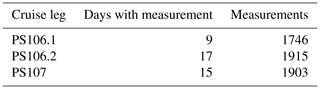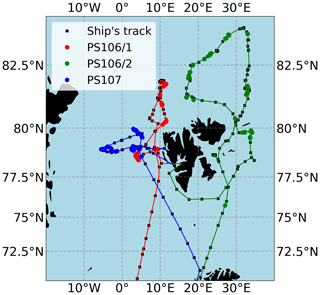Figure 1Map of the measurement area. Red markers indicate measurements during PS106.1 (24 May 2017 until 21 June 2017); green markers indicate measurements during PS106.2 (23 June 2017 until 19 July 2017). Blue markers indicate measurements during PS107 (22 July 2017 until 19 August 2017). The black squares show the ship's track.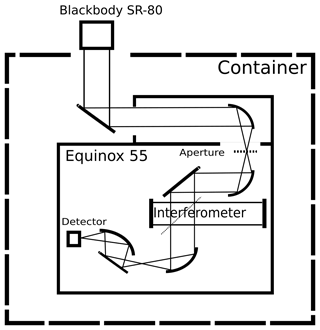Figure 2Sketch of IFS 55 Equinox. The blackbody SR-80 can be removed; then atmospheric radiation is measured.

2 Observations

## 2.1 Area of measurements

Measurements were performed around Svalbard from the 24 May 2017 until the 19 August 2017 within the scope of the cruise legs PS106.1 (PASCAL), PS106.2 (SiPCA) and PS107 (FRAM), performed by RV Polarstern. PS106.1 and PS106.2 are collectively referred to as PS106. The cloud cover was observed by meteorologists of the German Weather Service, who reported a cloud coverage of 7 or 8 oktas for approximately 75 % of the time. For further descriptions refer to and . Figure 1 shows the positions of the measurement sites and the ship.

## 2.2 Measurement setup

Measurements of the atmospheric radiances are performed with a mobile FTIR spectrometer (IFS 55 Equinox by Bruker Optics GmbH) in emission mode (measures atmospheric radiation without an external light source), which will from now on be referred to as EM-FTIR. The instrument was located in an air-conditioned and insulated container on the A-Deck of RV Polarstern. The roof of the container has two openings. The EM-FTIR was located below one opening. Both openings can be closed in case of precipitation. The interferometer inside the FTIR spectrometer has a movable mirror that gives a maximum optical path difference of 3 cm, which results in a maximum spectral resolution of $\mathrm{\Delta }\overline{\mathit{\nu }}=\mathrm{0.3}\phantom{\rule{0.125em}{0ex}}{\mathrm{cm}}^{-\mathrm{1}}$. To prevent damage from the hygroscopic substance of the beam splitter (potassium bromide), the spectrometer is permanently purged with dry air. Further specifications are described in Table 2. A blackbody (SR-80 by CI Systems) is placed manually on the EM-FTIR opening at regular intervals to perform a radiometric calibration.

$\begin{array}{}\text{(1)}& B=\mathit{\epsilon }B\left({T}_{\mathrm{BB}}\right)+\left(\mathrm{1}-\mathit{\epsilon }\right)B\left({T}_{\mathrm{lab}}\right),\end{array}$

with the temperature of the blackbody TBB and the temperature of the laboratory Tlab weighted by the blackbody emissivity ε .

The radiometric calibration of the spectrometer is performed using

$\begin{array}{}\text{(2)}& \begin{array}{rl}{L}_{\mathrm{atm}}& =\mathit{\epsilon }{B}_{\overline{\mathit{\nu }}}\left({T}_{\mathrm{amb}}\right)+\mathit{\epsilon }\frac{{B}_{\overline{\mathit{\nu }}}\left({T}_{\mathrm{hot}}\right)-{B}_{\overline{\mathit{\nu }}}\left({T}_{\mathrm{amb}}\right)}{\mathcal{F}\left({I}_{\mathrm{hot}}-{I}_{\mathrm{amb}}\right)}\\ & \cdot \mathcal{F}\left({I}_{\mathrm{atm}}-{I}_{\mathrm{amb}}\right)+\left(\mathrm{1}-\mathit{\epsilon }\right){B}_{\overline{\mathit{\nu }}}\left({T}_{\mathrm{lab}}\right).\end{array}\end{array}$

B(Tamb), B(Thot) and B(Tlab) are the Planck functions at high temperature (Thot, set to 100 C), surface air temperature (Tamb) and the temperature of the laboratory (Tlab) respectively. Ihot, Iamb and Iatm are the interferograms of the hot blackbody, blackbody at ambient temperature and atmospheric measurement respectively. is the operator for the Fourier transform. In contrast to the procedure described in , here the difference in the interferograms is calculated before applying the Fourier transform.

The following cycle is applied for the radiometric calibration: blackbody at Thot, atmospheric radiation, blackbody at Tamb, atmospheric radiation, blackbody at Thot and so on. Each measurement cycle of the blackbodies took about 10 min to obtain one blackbody interferogram Ihot or Iamb. The duration of the atmospheric measurements was approximately 15 min. The measurement time and schedule are chosen based on the time it took the blackbody to reach the desired temperature.

## 2.4 OCEANET measurements and Cloudnet synergistic retrieval

Retrievals of microphysical cloud parameters are compared with results of the synergistic retrieval Cloudnet. The OCEANET-Atmosphere observatory of the Leibniz Institute for Tropospheric Research (TROPOS) in Leipzig (Germany) performed continuous measurements during PS106.1 and PS106.2 . Its container houses a multi-wavelength Raman polarization lidar PollyXT and a microwave radiometer Humidity and Temperature Profiler (HATPRO), which were complemented during PS106 by a vertically pointing motion-stabilized 35 GHz cloud radar Mira-35. The OCEANET measurements provide profiles of aerosol and cloud properties and column-integrated liquid water and water vapor content. To retrieve products like liquid water content (LWC) and ice water content (IWC), the instrument synergistic approach Cloudnet was applied to these observations. The retrieved Cloudnet dataset during PS106 has been made available via PANGAEA (see Table 7). As atmospheric input, radiosondes launched from RV Polarstern were used. If no radiosonde was available, radiosondes from Ny-Ålesund (if the ship was near Svalbard) or model data from the Global Data Assimilation System model (GDAS1) were used. A short summary of the Cloudnet retrieval is given in Appendix A. For a detailed description please refer to and the publications cited there.

3 Atmospheric profiles and cloud height information

Auxiliary data obtained in the ship cruise itself were used to construct the atmospheric setup used in the retrieval. These include temperature and humidity profiles as well as cloud ceiling measurements.

## 3.1 Cloud ceiling

Information about the cloud ceiling was obtained using a Vaisala CL51 ceilometer operated by the German Weather Service. The maximum cloud detection altitude is 13 km with a vertical resolution of 10 m. The uncertainty in the retrieved ceiling is ±1 % but at least ±5m. The temporal resolution of the results is 60 s. Although only data of the cloud base height are given, it was decided to use these data instead of the Cloudnet height profile because the ceilometer data were available during the entire cruise, whereas the Cloudnet measurements were only available for PS106. Without changing the input data, a consistent dataset for the retrieval should be created. However, there is a mean bias between the cloud base height stated by Cloudnet and the ceilometer of −639m (median bias of −47m), which means on average the Cloudnet cloud base height is larger than the ceiling given by the ceilometer, and a root-mean-square error of 1870 m. Data of the ceilometer are available at .

Radiosondes were launched four times per day (00:00, 06:00, 12:00, 18:00 UTC) during PS106 and twice per day (06:00 and 12:00 UTC) during PS107 (Schmithüsen2017d, e, f). Data were measured using an RS92 radiosonde by Vaisala. Data of the air pressure, temperature, relative humidity, wind speed and wind direction were recorded. Accuracies are 0.5 K for temperature measurements, 5 % for relative humidity and 1 hPa for air pressure. Only atmospheric pressure, temperature and humidity were used here. Atmospheric profiles between two radiosonde launches are acquired by linear interpolation. If a radiosonde stopped measurements before reaching 30 km, data were extended using the ERA5 reanalysis .

4 Total Cloud Water retrieval (TCWret)

Total Cloud Water retrieval (TCWret) is a retrieval algorithm for microphysical cloud parameters from FTIR spectra. It is inspired by MIXCRA (Turner2005) and XTRA and uses an optimal estimation approach (Rodgers2000) to invert the measured spectral radiances for retrieving microphysical cloud parameters. For a complete description of the retrieval, please refer to Appendix B.

Two radiative transfer models are used in TCWret: the LBLRTM and DISORT . DISORT is called by LBLDIS (Turner2005) to calculate spectral radiances.

The LBLRTM calculates the optical depth for gaseous absorbers and the water vapor continuum. Either the profiles of H2O, CO2, O3, CO, CH4 and N2O can be set by the user, or a predefined atmosphere is used. A subarctic summer atmosphere, implemented in the LBLRTM, has been used for all gases except H2O, which has been read from radiosonde measurements.

DISORT calculates the monochromatic radiative transfer through a vertically inhomogeneous plane-parallel medium including scattering, absorption and emission. It provides the spectral radiances using single-scatter parameters.

Several databases are included in LBLDIS (Turner2014). These databases contain extinction cross sections, absorption cross sections, scattering cross sections, single-scattering albedo, the asymmetry factor and phase functions for different wavenumbers and effective radii. Refractive indices for liquid water droplets and ice crystals are taken from and respectively. Temperature-dependent refractive indices for liquid water are from . However, it is important to note that they have large uncertainties from 1000 to 1300 cm−1 . Scattering properties for more complex ice particle shapes like aggregates, bullet rosettes, droxtals, hollow columns, solid columns, plates and spheroids were calculated by using a combination of finite-difference time domain (FDTD), geometric optics and Mie theory.

For all liquid droplets and ice crystals, the droplet size distributions follow a gamma size distribution. The gamma size distributions were chosen in a way that they fit to the data during the First International Satellite Cloud Climatology Project (ISCCP) Regional Experiment (FIRE) Arctic Cloud Experiment (ACE). For further details, please refer to .

## 4.2 Products of TCWret

Direct retrieval products are τliq, τice, rliq and rice. From these parameters the water paths are calculated:

$\begin{array}{}\text{(3)}& \text{LWP}=\frac{\mathrm{2}}{\mathrm{3}}\cdot {r}_{\mathrm{liq}}\cdot {\mathit{\tau }}_{\mathrm{liq}}\cdot {\mathit{\varrho }}_{\mathrm{liq}}\phantom{\rule{0.25em}{0ex}},\text{(4)}& \text{IWP}=\frac{N\cdot {V}_{\mathrm{0}}\left({r}_{\mathrm{ice}}\right)\cdot {\mathit{\tau }}_{\mathrm{ice}}}{{\mathit{\alpha }}_{\mathrm{ice}}}\cdot {\mathit{\varrho }}_{\mathrm{ice}}\phantom{\rule{0.25em}{0ex}},\end{array}$

with the volumetric mass densities of liquid water ${\mathit{\varrho }}_{\mathrm{liq}}=\mathrm{1000}\phantom{\rule{0.125em}{0ex}}\mathrm{kg}\phantom{\rule{0.125em}{0ex}}{\mathrm{m}}^{-\mathrm{3}}$ and ice water ${\mathit{\varrho }}_{\mathrm{ice}}=\mathrm{917}\phantom{\rule{0.125em}{0ex}}\mathrm{kg}\phantom{\rule{0.125em}{0ex}}{\mathrm{m}}^{-\mathrm{3}}$, the particle number density N, and the extinction coefficient ${\mathit{\alpha }}_{\mathrm{ice}}={\mathit{\beta }}_{\mathrm{ice}}\cdot N$. The total volume of an ice crystal V0(rice) and the extinction cross section of an ice droplet βice, both integrated over the gamma size distribution, are read from the databases of single-scattering parameters. The formula for the liquid water path works for spherical droplets only, while the formula for the ice water path is valid for ice crystals of any shape (Turner2005). The covariance matrix Sr of the optimal estimation procedure is used to determine the errors.

## 4.3 Covariance matrix and averaging kernels

Retrieval errors are calculated from the variance–covariance matrix Sr of the retrieval. It is calculated by

$\begin{array}{}\text{(5)}& {\mathbf{S}}_{\mathrm{r}}={\mathbf{T}}_{\mathrm{r}}{\mathbf{S}}_{y}{\mathbf{T}}_{\mathrm{r}}^{T}.\end{array}$

The index r denotes quantities of the final iteration. T is a transfer matrix, and Sy is the variance–covariance matrix of the measurement. The retrieval uses a Levenberg–Marquardt algorithm; therefore the variance–covariance matrix and the transfer matrix T are calculated iteratively, as described by . Another important quantity to characterize the retrieval quality is the averaging kernel matrix A. The averaging kernel matrix contains the derivatives of the retrieved quantities with respect to the true state vector:

$\begin{array}{}\text{(6)}& \mathbf{A}=\frac{\partial {\mathbit{x}}_{\mathrm{r}}}{\partial {\mathbit{x}}_{\mathrm{t}}},\end{array}$

where xr means the retrieved parameters and xt denotes the unknown true parameters. In TCWret, the averaging kernel matrix is a 4×4 matrix. The top two rows belong to τliq and τice; the bottom two rows belong to rliq and rice. On the diagonal elements one finds the derivatives of each element in the retrieved state vector with respect to its corresponding element in the true state vector. Off-diagonal elements give the degree of correlation between the entries of the state vector:

$\begin{array}{}\text{(7)}& \mathbf{A}=\left(\begin{array}{cccc}{A}_{{\mathit{\tau }}_{\mathrm{liq}}}& {A}_{{\mathit{\tau }}_{\mathrm{liq}},{\mathit{\tau }}_{\mathrm{ice}}}& {A}_{{\mathit{\tau }}_{\mathrm{liq}},{r}_{\mathrm{liq}}}& {A}_{{\mathit{\tau }}_{\mathrm{liq}},{r}_{\mathrm{ice}}}\\ {A}_{{\mathit{\tau }}_{\mathrm{ice}},{\mathit{\tau }}_{\mathrm{liq}}}& {A}_{{\mathit{\tau }}_{\mathrm{ice}}}& {A}_{{\mathit{\tau }}_{\mathrm{ice}},{r}_{\mathrm{liq}}}& {A}_{{\mathit{\tau }}_{\mathrm{ice}},{r}_{\mathrm{ice}}}\\ {A}_{{r}_{\mathrm{liq}},{\mathit{\tau }}_{\mathrm{liq}}}& {A}_{{r}_{\mathrm{liq}},{\mathit{\tau }}_{\mathrm{ice}}}& {A}_{{r}_{\mathrm{liq}}}& {A}_{{r}_{\mathrm{liq}},{r}_{\mathrm{ice}}}\\ {A}_{{r}_{\mathrm{ice}},{\mathit{\tau }}_{\mathrm{liq}}}& {A}_{{r}_{\mathrm{ice}},{\mathit{\tau }}_{\mathrm{ice}}}& {A}_{{r}_{\mathrm{liq}},{r}_{\mathrm{ice}}}& {A}_{{r}_{\mathrm{ice}}}\end{array}\right).\end{array}$

Here Av,w stands for the mutual dependence of the parameters v and w, where v is the parameter in xr and w is the parameter in xt. The trace of the averaging kernel matrix gives the degrees of freedom of the signal, which can be interpreted as the number of individually retrievable parameters from the measurement (Rodgers2000). The averaging kernel matrix sets the retrieval and the a priori into context:

$\begin{array}{}\text{(8)}& {\mathbit{x}}_{\mathrm{r}}={\mathbit{x}}_{\mathrm{a}}+\mathbf{A}\left({\mathbit{x}}_{\mathrm{t}}-{\mathbit{x}}_{\mathrm{a}}\right).\end{array}$

From this relationship it can be seen that in the optimal case the averaging kernel matrix is the unit matrix. Smaller entries mean a stronger influence by the a priori. Averaging kernels in TCWret are calculated via

$\begin{array}{}\text{(9)}& \mathbf{A}={\mathbf{T}}_{\mathrm{r}}{\mathbf{K}}_{\mathrm{r}}\phantom{\rule{0.25em}{0ex}}.\end{array}$

The matrix Kr is the Jacobian matrix of the retrieved parameters . Uncertainties in the LWP and IWP are calculated from error propagation:

$\begin{array}{}\text{(10)}& {\mathit{\sigma }}_{Y}=±\sqrt{\sum _{i}{\left(\frac{\partial Y}{\partial {m}_{i}}{\mathit{\sigma }}_{{m}_{i}}\right)}^{\mathrm{2}}},\end{array}$

where Y is either the LWP or the IWP; $\frac{\partial Y}{\partial m}$ is the partial derivative of Y with respect to an atmospheric parameter $m=\left\{{\mathit{\tau }}_{\mathrm{liq}},{\mathit{\tau }}_{\mathrm{ice}},{r}_{\mathrm{liq}},{r}_{\mathrm{ice}}\right\}$; and ${\mathit{\sigma }}_{{m}_{i}}$ is the variance of the ith parameter mi, as stated in Sr.

Table 3Results of the test case retrievals. $|r|$ is the correlation coefficient of each quantity. Mean bias is the mean difference between the retrieval and the true size of the parameter. RMSE is the root-mean-square error of the difference between the retrieval and true parameter. For τliq,ice and rliq,ice, ERR(OE) is the standard deviation calculated from the posterior covariance matrix of the optimal estimation, stated in Eq. (5). For the other quantities, ERR(OE) is calculated by error propagation. Maximum of quantity in test cases specifies the maximum value that can be used for this quantity in the test cases. A total number of 253 test cases are included in these calculations.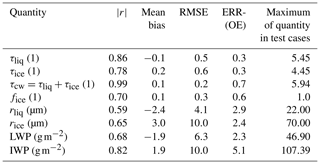## 4.4 Performance of TCWret applying to simulated data

In addition to the uncertainties indicated by the optimal estimation procedure, TCWret was applied to simulated data . The description of the test cases and the evaluation can be found in the Appendix C. Results are shown in Table 3. When applied to the simulated data, it could be shown that TCWret can determine all variables entered in the table. Results calculated by TCWret are comparable to the true cloud parameters from the simulated data.

## 4.5 Errors in atmospheric profile and calibration

Besides the uncertainties from the optimal estimation algorithm, uncertainties from atmospheric profile data and the calibration cycle increase the total uncertainty in the data.

Table 4Mean partial derivatives, used for estimating the parameter errors Δm.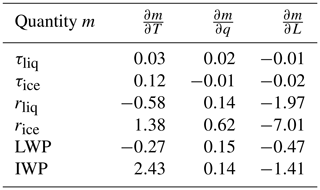### 4.5.1 Partial derivatives for non-retrieved quantities

To estimate the uncertainty which comes from the cloud temperature, humidity profile and spectral calibration, the test cases from have been adjusted to incorporate uncertainties in cloud temperature, humidity and radiance. Three datasets are created, each of them with one of the following adjustments:

• increase cloud temperature by 1 K

• increase atmospheric humidity by 10 %

• increase radiance by $\mathrm{2}\phantom{\rule{0.125em}{0ex}}\mathrm{mW}\phantom{\rule{0.125em}{0ex}}\left({\mathrm{m}}^{\mathrm{2}}\phantom{\rule{0.125em}{0ex}}\mathrm{sr}\phantom{\rule{0.125em}{0ex}}{\mathrm{cm}}^{-\mathrm{1}}{\right)}^{-\mathrm{1}}$.

With these datasets the partial derivatives are calculated, which are necessary to determine the errors due to cloud temperature, humidity and spectral calibration and propagate them into the retrieved cloud parameters by application of

$\begin{array}{}\text{(11)}& \mathrm{\Delta }m=±\sqrt{{\left(\frac{\partial m}{\partial T}\mathrm{\Delta }T\right)}^{\mathrm{2}}+{\left(\frac{\partial m}{\partial q}\mathrm{\Delta }q\right)}^{\mathrm{2}}+{\left(\frac{\partial m}{\partial L}\mathrm{\Delta }L\right)}^{\mathrm{2}}},\end{array}$

with the cloud temperature T; the relative humidity q; the radiance L; and their errors ΔT, Δq and ΔL. To separate the influence of the parameter errors from the retrieval performance, the results of these three datasets are compared to the retrieval results mentioned in Sect. 4.4 instead of the true cloud parameters. Mean partial derivatives are then calculated as follows:

1. Retrieve the cloud parameters for each dataset.

2. Calculate the difference between the cloud parameters of the adjusted dataset and the undisturbed dataset (which has been already used in Sect. 4.3).

3. Calculate the difference quotients, which will act as partial derivatives in Eq. (11).

The partial derivatives are shown in Table 4.

### 4.5.2 Temperature and humidity

Device errors in the radiosonde are ΔT=0.5 K and Δq=5 %. Additionally, the error introduced with the linear interpolation of the temperature and relative humidity is estimated by comparing the interpolated profiles to atmospheric profiles from ERA5. The interpolation error follows from the comparison between the linear interpolation between two radiosonde measurements and the ERA5 atmosphere at the position of the measurements. We query the ERA5 atmosphere for each hour. Then we calculate the atmospheric profiles from the radiosondes once per hour by linear interpolation. From this we calculate the difference, average over 1 d and calculate the standard deviation. Figure 4 gives the total error as device error and interpolation error, as an example for the period from 11 June 2017 to 30 June 2017.

### 4.5.3 Calibration error

The accuracy of the blackbody temperature and emissivity are $\mathrm{\Delta }{T}_{\mathrm{BB}}=±\mathrm{0.05}\phantom{\rule{0.125em}{0ex}}\mathrm{K}$ and $\mathrm{\Delta }\mathit{ϵ}=±\mathrm{0.02}$. The propagation of these errors into the radiance is

$\begin{array}{}\text{(12)}& \mathrm{\Delta }L=\sqrt{{\left(\frac{\partial {L}_{\mathrm{atm}}}{\partial \mathit{ϵ}}\cdot \mathrm{0.02}\right)}^{\mathrm{2}}+{\left(\frac{\partial {L}_{\mathrm{atm}}}{\partial {T}_{\mathrm{BB}}}\cdot \mathrm{0.05}\phantom{\rule{0.125em}{0ex}}\mathrm{K}\right)}^{\mathrm{2}}}.\end{array}$

To estimate $\frac{\partial {L}_{\mathrm{atm}}}{\partial \mathit{ϵ}}$, a spectrum is calibrated with an emissivity of ϵ and ${\mathit{ϵ}}^{\prime }+h$. The partial derivative is calculated by $\frac{\partial {L}_{\mathrm{atm}}}{\partial \mathit{ϵ}}=\frac{L\left({\mathit{\epsilon }}^{\prime }+h\right)-L\left({\mathit{\epsilon }}^{\prime }\right)}{h}$ with L(ε) being the radiance under the emissivity ε and h as the step size for the numerical calculation of the partial derivative. From ${\mathit{ϵ}}^{\prime }=\mathrm{0.975}$ and h=0.02 follows $\frac{\partial {L}_{\mathrm{atm}}}{\partial \mathit{ϵ}}\cdot \mathrm{0.02}=-\mathrm{0.98}\phantom{\rule{0.125em}{0ex}}\mathrm{mW}\phantom{\rule{0.125em}{0ex}}\left({\mathrm{m}}^{\mathrm{2}}\phantom{\rule{0.125em}{0ex}}\mathrm{sr}\phantom{\rule{0.125em}{0ex}}{\mathrm{cm}}^{-\mathrm{1}}{\right)}^{-\mathrm{1}}$. The second partial derivative $\frac{\partial {L}_{\mathrm{atm}}}{\partial {T}_{\mathrm{BB}}}$ is estimated using Eq. (2). The emissivity is set to 1. The measured radiance of the hot blackbody is larger than the radiance of the atmosphere (ℱ(Ihot)>ℱ(Iatm)), and therefore the quotient

$\begin{array}{}\text{(13)}& \frac{\mathcal{F}\left({I}_{\mathrm{atm}}-{I}_{\mathrm{amb}}\right)}{\mathcal{F}\left({I}_{\mathrm{hot}}-{I}_{\mathrm{amb}}\right)}<\mathrm{1}.\end{array}$

From the measurements it follows that Lhot is about 5 times larger than Lamb; therefore the inequation (Eq. 13) is set as $\frac{\mathcal{F}\left({I}_{\mathrm{hat}}-{I}_{\mathrm{amb}}\right)}{\mathcal{F}\left({I}_{\mathrm{hot}}-{I}_{\mathrm{amb}}\right)}=\mathrm{0.2}$. Equation (2) thus can be written as

$\begin{array}{}\text{(14)}& {L}_{\mathrm{atm}}={B}_{\overline{\mathit{\nu }}}\left({T}_{\mathrm{amb}}\right)+\mathrm{0.2}\cdot {B}_{\overline{\mathit{\nu }}}\left({T}_{\mathrm{hot}}\right)-{B}_{\overline{\mathit{\nu }}}\left({T}_{\mathrm{amb}}\right).\end{array}$

With ${T}_{\mathrm{BB}}={T}_{\mathrm{hot}}=\mathrm{100}$C and Tamb=0C, $\frac{\partial {L}_{\mathrm{atm}}}{\partial {T}_{\mathrm{BB}}}\cdot \mathrm{0.05}=\mathrm{0.10}\phantom{\rule{0.125em}{0ex}}\mathrm{mW}\phantom{\rule{0.125em}{0ex}}\left({\mathrm{m}}^{\mathrm{2}}\phantom{\rule{0.125em}{0ex}}\mathrm{sr}\phantom{\rule{0.125em}{0ex}}{\mathrm{cm}}^{-\mathrm{1}}{\right)}^{-\mathrm{1}}$ is an average for the spectral interval between 500 and 2000 cm−1. This gives $\mathrm{\Delta }L=\mathrm{0.98}\phantom{\rule{0.125em}{0ex}}\mathrm{mW}\phantom{\rule{0.125em}{0ex}}\left({\mathrm{m}}^{\mathrm{2}}\phantom{\rule{0.125em}{0ex}}\mathrm{sr}\phantom{\rule{0.125em}{0ex}}{\mathrm{cm}}^{-\mathrm{1}}{\right)}^{-\mathrm{1}}$.

### 4.5.4 Resulting parameter error

Finally, from the calculations in this section, the resulting uncertainties are as follows:

• ΔT=2.0 K, as the sum of the device error (0.5 K) and the interpolation error (1.5 K).

• Δq=17.5 %, as the sum of the device error (5.0 %) and the interpolation error (12.5 %).

• $\mathrm{\Delta }L=\mathrm{0.98}\phantom{\rule{0.125em}{0ex}}\mathrm{mW}\phantom{\rule{0.125em}{0ex}}\left({\mathrm{m}}^{\mathrm{2}}\phantom{\rule{0.125em}{0ex}}\mathrm{sr}\phantom{\rule{0.125em}{0ex}}{\mathrm{cm}}^{-\mathrm{1}}{\right)}^{-\mathrm{1}}$.

Applying these uncertainties to Eq. (11), the uncertainties for each parameter are Δτliq=0.4, Δτice=0.3, Δrliq=3.3 µm, Δrice=13.1 µm, $\mathrm{\Delta }\text{LWP}=\mathrm{2.8}\phantom{\rule{0.125em}{0ex}}\mathrm{g}\phantom{\rule{0.125em}{0ex}}{\mathrm{m}}^{-\mathrm{2}}$ and $\mathrm{\Delta }\text{IWP}=\mathrm{5.6}\phantom{\rule{0.125em}{0ex}}\mathrm{g}\phantom{\rule{0.125em}{0ex}}{\mathrm{m}}^{-\mathrm{2}}$. These values will be added to the retrieval errors in the next section.Figure 5Distribution of retrieved optical depths for liquid water (a) and ice water (b). The bin width is set to the sum of the root-mean-square error from Table 3 and the errors discussed in Sect. 4.5.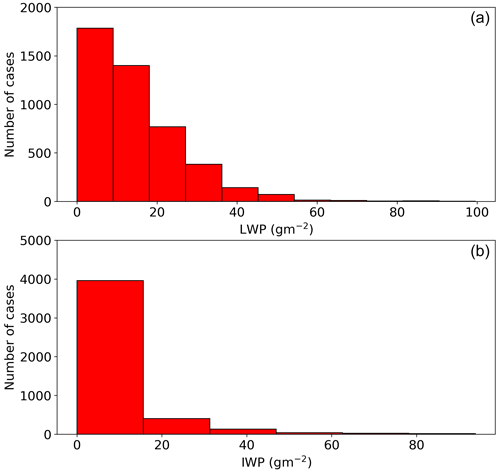Figure 6Distribution of the retrieved LWP (a) and IWP (b). The bin width is set to the sum of the root-mean-square error from Table 3 and the errors discussed in Sect. 4.5.Figure 7Distribution of retrieved effective radii for liquid water droplets (a) and ice crystals (b). The bin width is set to the sum of the root-mean-square error from Table 3 and the errors discussed in Sect. 4.5. In each instance, only cases are considered in which the phase fractions are above 0.1 (liquid water fraction for rliq and ice water fraction for rice). This results in 4111 of 4590 cases for rliq (89.6 %) and 2153 of 4590 cases for rice (46.9 %).

5 Results

## 5.1 Cloud parameters from infrared radiance measurements during PS106 and PS107

During the measurement campaign, most of the observed optical depth is due to liquid water instead of ice crystals. Histograms of all retrieved optical depths are shown in Fig. 5. In 66.4 % of the measurements, ice was observed in the clouds, whereas in 92.4 % of the measurements liquid water was present. Mean optical depths are τliq=2.6 and τice=0.8. Similarly to the optical depth, most of the observed cloud water is liquid water (Fig. 6). Here the means are $\text{LWP}=\mathrm{17.7}\phantom{\rule{0.125em}{0ex}}\mathrm{g}\phantom{\rule{0.125em}{0ex}}{\mathrm{m}}^{-\mathrm{2}}$ and $\text{IWP}=\mathrm{9.9}\phantom{\rule{0.125em}{0ex}}\mathrm{g}\phantom{\rule{0.125em}{0ex}}{\mathrm{m}}^{-\mathrm{2}}$. Interquartile ranges for the LWP and IWP are ${\mathrm{IQR}}_{\mathrm{LWP}}=\mathrm{18.9}\phantom{\rule{0.125em}{0ex}}\mathrm{g}\phantom{\rule{0.125em}{0ex}}{\mathrm{m}}^{-\mathrm{2}}$ and ${\mathrm{IQR}}_{\mathrm{IWP}}=\mathrm{11.5}\phantom{\rule{0.125em}{0ex}}\mathrm{g}\phantom{\rule{0.125em}{0ex}}{\mathrm{m}}^{-\mathrm{2}}$. Whereas the range of the LWP matches the LWP from the test cases, the IWP is near the lower threshold of the retrievable water path.

The distributions of the effective radii are shown in Fig. 7. For rliq only cases with fice<0.9 are used, and for rice only cases with fice>0.1 are used. On average, ice crystals (rice=22.3 µm) are larger than liquid droplets (rliq=10.9 µm). Ice crystals show a wider range of retrieved effective radii than liquid droplets, expressed by an interquartile range of IQRice=17.9 µm compared to IQRliq=5.9 µm.

## 5.2 Averaging kernels and posterior correlation matrices

For all measurements, the mean of the averaging kernels and degrees of freedom are calculated:

$\begin{array}{}\text{(15)}& \mathbf{A}& =\left(\begin{array}{cccc}\mathrm{0.77}& \mathrm{0.48}& -\mathrm{0.17}& -\mathrm{0.02}\\ \mathrm{0.19}& \mathrm{0.45}& \mathrm{0.25}& -\mathrm{0.01}\\ -\mathrm{0.04}& \mathrm{0.14}& \mathrm{0.74}& \mathrm{0.05}\\ -\mathrm{0.03}& -\mathrm{0.1}& \mathrm{0.29}& \mathrm{0.3}\end{array}\right),\text{(16)}& tr\left(\mathbf{A}\right)& =\mathrm{2.25}.\end{array}$

This mean averaging kernel matrix contains both single-phase clouds and mixed-phase clouds. Since only two parameters are determined in the single-phase cases, they perturb the mean number of degrees of freedom for all measurements. As seen in the statistics, there are fewer cases with ice-containing clouds. This lowers the entries on the diagonals for τice and rice as they are 0 in all-liquid clouds. Therefore, the mean averaging kernel was also calculated for all mixed-phase clouds:

$\begin{array}{}\text{(17)}& {\mathbf{A}}_{\text{mixed-phase}}=\left(\begin{array}{cccc}\mathrm{0.62}& \mathrm{0.22}& -\mathrm{0.35}& -\mathrm{0.03}\\ \mathrm{0.32}& \mathrm{0.7}& \mathrm{0.47}& -\mathrm{0.04}\\ -\mathrm{0.08}& \mathrm{0.16}& \mathrm{0.66}& \mathrm{0.1}\\ -\mathrm{0.14}& -\mathrm{0.07}& \mathrm{0.17}& \mathrm{0.59}\end{array}\right)\text{(18)}& tr\left({\mathbf{A}}_{\text{mixed-phase}}\right)=\mathrm{2.57}\end{array}$

The number of degrees of freedom in this case is 2.57. The entries for the effective radii are of the same size as those for the optical depth.

The posterior correlation matrix R gives the correlations of one retrieved parameter to another. For mixed-phase clouds, R is as follows:

$\begin{array}{}\text{(19)}& {\mathbf{R}}_{\text{mixed-phase}}=\left(\begin{array}{cccc}\mathrm{1.00}& \mathrm{0.50}& -\mathrm{0.07}& -\mathrm{0.40}\\ \mathrm{0.50}& \mathrm{1.00}& \mathrm{0.02}& -\mathrm{0.23}\\ -\mathrm{0.07}& \mathrm{0.02}& \mathrm{1.00}& \mathrm{0.13}\\ -\mathrm{0.41}& -\mathrm{0.23}& \mathrm{0.13}& \mathrm{1.00}\end{array}\right).\end{array}$

The largest correlation appears between τliq and τice ($|r|=\mathrm{0.50}$), which points to a difficult phase determination. Apart from the correlation of the optical depths, the comparatively high correlation between rice and τliq is striking, which suggests that both parameters cannot be determined completely independently of each other.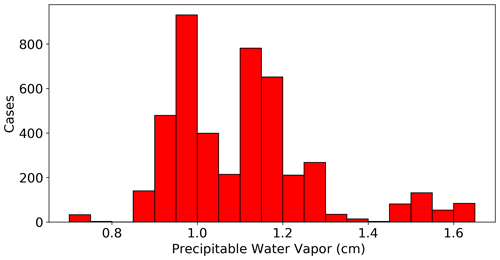Figure 8Histogram of the precipitable water vapor during the measurements of atmospheric radiances.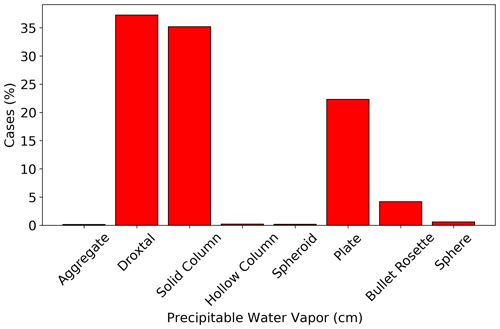Figure 9Percentage of retrievals for each ice particle shape. Most particles are modeled as droxtals (37 %), solid columns (35 %), plates (22 %) and bullet rosettes (4 %).

## 5.3 Precipitable water vapor

A crucial spectral region for the determination of the cloud phase is the spectral window in the far infrared between 500 and 600 cm−1 . This spectral region is sensitive to the concentration of water vapor in the atmosphere. The amount of water vapor is expressed by the precipitable water vapor PWV, which has been calculated from the radiosonde measurements. The far-infrared spectral region becomes nearly opaque to infrared radiation for PWV >1 cm . During the measurement campaign the PWV was greater than 1 cm in 62 % of the cases. Therefore, the datasets for PWV greater than 1 cm are not removed from the analysis. Statistics of PWV are shown in Fig. 8.

Table 6Results of the comparison between TCWret and Cloudnet. Mean bias and root-mean-square error refer to the difference in both datasets.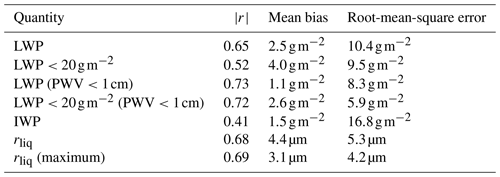## 5.4 Comparison to Cloudnet

To compare results from TCWret and Cloudnet, a combined dataset of TCWret results is created in the following way. Since the shapes of the ice crystals are not known, the retrievals were carried out for all ice crystal shapes. However, this procedure leads to up to eight results per measurement, so a selection was made. The aim of the following selection is that all ice crystals with rice<30 µm are modeled as droxtals, while larger ice crystals are modeled as plates, bullet rosettes or solid columns. This choice is motivated by . The accepted result is then determined as follows:

1. If rice for plates, bullet rosettes and solid columns or for droxtals is less than 30 µm, the result using ice crystals as droxtals is accepted.

2. If rice for droxtals is greater than 10 µm, the result that uses plates, bullet rosettes or solid columns is accepted. To choose one of the datasets, a random number is drawn which selects plates in 35 %, bullet rosettes in 15 % and solid columns in 50 % of cases.

3. If none of the conditions apply, the data for which the degrees of freedom of the outcome are highest are accepted.

The first condition ensures that all small ice particles are classified as droxtals, while the second ensures that all larger particles are classified as plates, solid columns or bullet rosettes. Stricter thresholds would more often result in only the last condition applying, which should be avoided as much as possible.

As an additional constraint, we only allow results where rliq<rice. This is motivated by the following: the results of rliq and rice show that rliq is usually smaller than rice. This applies to both TCWret and Cloudnet. Therefore, cases with rliq>rice are likely cases with a too small rice and a too large rliq. For the comparison between TCWret and Cloudnet, results from both datasets were averaged over a time period of 2 min. This has been done because the underlying measurement systems have different temporal resolutions; also both measurement systems were at different locations on the ship. Cloudnet results do not contain optical depths but water paths and droplet radii; therefore we will compare the LWP and IWP, rliq and rice. Correlation coefficients, mean biases and root-mean-square errors are shown in Table 6.

### 5.4.1 Ice water path and ice effective radius

Although TCWret can determine rice from the simulated spectra, no correlation can be found between the TCWret and Cloudnet data. From the error considerations in previous sections it was shown that the RMSE for the simulated spectra is already 10.0 µm. Taking into account uncertainties in the atmospheric data and the calibration, an additional uncertainty term of 13.1 µm is obtained, so rice is already subject to high uncertainties. According to the posterior correlation matrix, rice correlates with τliq, so there is no completely independent result of rice. A better determination of rice could be achieved by a better a priori xa, but the problem remains that according to the averaging kernel matrix, only 2.57 degrees of freedom exist in the measurements.

Figure 10 shows the results for the IWP. Although a correlation can be found, there is large spread between the datasets. The difference between TCWret and Cloudnet is $\left(\mathrm{1.5}±\mathrm{16.8}\right)\phantom{\rule{0.125em}{0ex}}\mathrm{g}\phantom{\rule{0.125em}{0ex}}{\mathrm{m}}^{-\mathrm{2}}$. The IWP is calculated according to Eq. (4) from τice and rice, where rice influences the IWP of the TCWret dataset. Furthermore, the IWP during the measurement campaign is 9.9 g m−2, very low and within the RMSE of TCWret when retrieving the simulated spectra. The IWP is therefore at the lower limit of what can be determined with TCWret and is considered less reliable.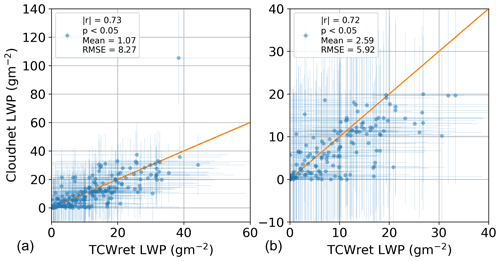Figure 11Liquid water path of TCWret versus Cloudnet for PWV<1 cm. Left scatterplot (a) contains all measurements, whereas the right plot (b) only shows clouds with LWP $<\mathrm{20}\phantom{\rule{0.125em}{0ex}}\mathrm{g}\phantom{\rule{0.125em}{0ex}}{\mathrm{m}}^{-\mathrm{2}}$.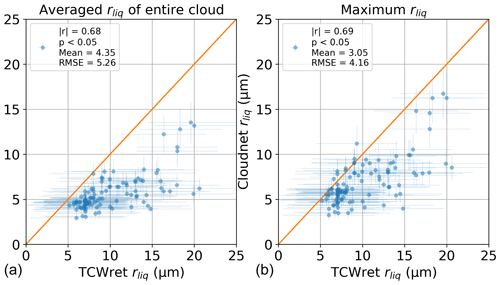Figure 12The rliq of TCWret versus rliq of Cloudnet averaged over the entire cloud (a) and maximum value of the cloud from Cloudnet (b).

### 5.4.2 Liquid water path and effective droplet radius

Results of the liquid water path from TCWret and Cloudnet are correlated. The difference is ($\mathrm{2.5}±\mathrm{10.4}\right)\phantom{\rule{0.125em}{0ex}}\mathrm{g}\phantom{\rule{0.125em}{0ex}}{\mathrm{m}}^{-\mathrm{2}}$ with no restriction to the maximum water path. From this we conclude that the LWP from the TCWret dataset is reliable. As mentioned earlier, a large PWV value interferes with the retrieval, as the water vapor has a larger influence on the microwindows. Therefore, we additionally remove all cases from the analysis where the PWV is larger than 1 cm. This reduces the mean bias to 1.1 g m−2 and the RMSE to 8.3 g m−2. The results with PWV <1 cm are shown in Fig. 11, left panel.

Since the LWP of TCWret correlates with that of the Cloudnet product and since the RMSE of the LWP is far below the uncertainty in the LWP of the Cloudnet product, we reduced the maximum LWP to investigate whether a correlation can also be observed for clouds with an $\text{LWP}<\mathrm{20}\phantom{\rule{0.125em}{0ex}}\mathrm{g}\phantom{\rule{0.125em}{0ex}}{\mathrm{m}}^{-\mathrm{2}}$. With a real uncertainty of $±\mathrm{20}\phantom{\rule{0.125em}{0ex}}\mathrm{g}\phantom{\rule{0.125em}{0ex}}{\mathrm{m}}^{-\mathrm{2}}$ the correlation is expected to disappear.

Results for very thin clouds and PWV <1 cm are shown in Fig. 11 (right side). Again, results are correlated. The RMSE for these clouds is 5.9 g m−2 with a mean bias of 2.6 g m−2. Without any restrictions on the PWV, there is an RMSE of 9.5 g m−2 and a mean bias of 4.0 g m−2. From the comparison with TCWret, it can be concluded that during this measurement campaign, Cloudnet's results for thin clouds with LWP $<\mathrm{20}\phantom{\rule{0.125em}{0ex}}\mathrm{g}\phantom{\rule{0.125em}{0ex}}{\mathrm{m}}^{-\mathrm{2}}$ are also reliable despite the stated error of 20.40 g m−2.

It should be noted that Cloudnet and TCWret use the atmospheric profiles from the radiosonde measurements carried out on RV Polarstern. Apart from that, however, both the measuring instruments and the retrievals are different. Furthermore, TCWret does not use information from Cloudnet as a priori. Since TCWret has also shown comparable agreement with the LWP of the simulated spectra in the test cases (mean bias is $-\mathrm{1.6}\phantom{\rule{0.125em}{0ex}}\mathrm{g}\phantom{\rule{0.125em}{0ex}}{\mathrm{m}}^{-\mathrm{2}}$; RMSE is 6.3 g m−2), it is to be expected that TCWret and thus also Cloudnet have independently determined the LWP correctly.

Figure 12 shows the results for rliq. The left panel shows the results where rliq of Cloudnet is averaged over the entire cloud. The right panel shows the maximum rliq of the cloud in the Cloudnet data. Only results from TCWret are considered if fice<0.9. As in the LWP, a correlation between the data can be observed. Overall, there is an overestimation of the rliq of TCWret by 4.4 µm on average. If only considering the maximum rliq in Cloudnet, the mean bias decreases to 3.1 µm. The same applies to the RMSE, which decreases from 5.3 to 4.2 µm. These results indicate that rliq in TCWret does not take into account the entire cloud, which is to be expected since the rliq in Cloudnet is determined using the altitude-resolved radar reflectivity, while TCWret uses the radiance of the clouds measured on the ground. However, the observed correlation allows a correction of rliq in TCWret as a function of rliq itself.

6 Code availability

The retrieval algorithm TCWret is available at https://doi.org/10.5281/ZENODO.4621127 (Richter2021a) with external subroutines at https://doi.org/10.5281/ZENODO.4618142 (Richter2021b) and https://doi.org/10.5281/ZENODO.4618106 (Richter2021c). Jupyter notebooks to perform the comparisons to Cloudnet are available at https://doi.org/10.5281/zenodo.6647314 (Richter2022).

7 Data availability

For accessibility of the datasets used and shown, see Table 7.

8 Summary and conclusion

A dataset of microphysical cloud parameters of optically thin clouds is presented. The measurements were carried out on the ship RV Polarstern in summer 2017 in the Arctic Ocean around Svalbard and in the Fram Strait.

Measurements were performed using a mobile FTIR spectrometer, operated in emission mode (EM-FTIR). A calibration of the EM-FTIR was performed with a blackbody radiator, whose temperature was alternately set to 100 C and ambient temperature. The spectrometer was operated in an air-conditioned container. Radiances between 500 and 2000 cm−1 were recorded.

The retrieval of cloud parameters is performed using the Total Cloud Water retrieval (TCWret) algorithm. TCWret uses the optimal estimation method to invert atmospheric radiances. The radiative transfer model used is LBLDIS, which utilizes optical depths of atmospheric trace gases calculated with the LBLRTM and then calculates the spectral radiances using DISORT. Single-scattering parameters for clouds are read from pre-calculated databases. Retrieval products are the optical depths of water and ice and the corresponding effective radii. From these products, the liquid water path and ice water path are calculated. TCWret also uses profiles of air pressure, humidity and temperature from measurements with Vaisala RS92 radiosondes and information about cloud height from measurements of the ceilometer CL51, which is on board RV Polarstern.

During the measurement campaign, a dataset with 5564 retrievals was created. A comparison to the simultaneously performed retrievals of the Cloudnet network on the Polarstern shows the following:

• The LWPs of both datasets are correlated. From this it is concluded that the retrieved LWP from TCWret is reliable. In addition, it could be shown using the TCWret dataset that during this measurement campaign the measurement data of thin clouds (LWP $<\mathrm{20}\phantom{\rule{0.125em}{0ex}}\mathrm{g}\phantom{\rule{0.125em}{0ex}}{\mathrm{m}}^{-\mathrm{2}}$) of the Cloudnet retrieval are also reliable despite the given error of 20 g m−2.

• As well as for the LWP, a correlation for rliq is observed. However, there is an increasing bias with increasing rliq. This can be corrected using the results from Cloudnet.

• Only a low correlation can be found for the IWP, and rice does not correlate. Therefore the IWP is considered to be less reliable than the liquid water products.

Despite the difficulty in determining the IWP and rice, this presented dataset is useful for downward cloud radiative flux calculations. Since TCWret determines the cloud parameters from the spectral radiance, the calculated cloud parameters are those that match the observed radiance. This is also true if the IWP and rice are affected by errors.

In summary, the dataset of cloud parameters and water paths from TCWret provides a helpful complement to the results of the LWP from Cloudnet but at the same time benefits from its rliq. Due to the consistent calculation of cloud parameters over the entire cruise, the results from TCWret additionally provide information about clouds during PS107, where only EM-FTIR measurements are available.

Appendix A: Brief description of the Cloudnet synergistic retrieval

The LWP is determined using the HATPRO MWR, which uses two frequency bands between 22.24 and 31.4 GHz and between 51.0 and 58.0 GHz. A statistical retrieval has been set up using radiosonde data from Ny-Ålesund, consistent with the procedure described in and leading to an RMSE of 22.4 g m−2. If a data point was classified as pure liquid, the effective radius of the cloud droplets was determined from the radar reflectivity and the LWP according to the retrieval of . The IWC was determined according to via an empirical formula from temperature and radar reflectivity. The IWP was determined by vertical integration of the IWC. The calculation of the IWP was carried out specifically for this study. The determination of rice is carried out analogously to the IWC from the radar reflectivity and the temperature by an empirical formula .

Appendix B: Description of TCWret

## B1 Working principle of TCWret

TCWret retrieves optical depths of liquid water and ice water and the effective radii of liquid water droplets and ice crystals from infrared spectral radiances. The retrieval of microphysical cloud parameters is a nonlinear problem, so an iterative algorithm is needed:

$\begin{array}{}\text{(B1)}& {\mathbit{x}}_{n+\mathrm{1}}={\mathbit{x}}_{n}+{\mathbit{s}}_{n}\phantom{\rule{0.25em}{0ex}}.\end{array}$

Here xn and xn+1 are the state vectors containing cloud parameters of the nth and (n+1)th steps and sn is the modification of the cloud parameters during the nth iteration. The state vector contains the optical depths and effective radii:

$\begin{array}{}\text{(B2)}& {\mathbit{x}}_{n}=\left(\begin{array}{c}{\mathit{\tau }}_{\mathrm{liq},n}\\ {\mathit{\tau }}_{\mathrm{ice},n}\\ {r}_{\mathrm{liq},n}\\ {r}_{\mathrm{ice},n}\end{array}\right).\end{array}$

The governing equation to determine sn is

$\begin{array}{}\text{(B3)}& \begin{array}{rl}& \left({\mathbf{K}}_{n}^{T}{{\mathbf{S}}_{\mathbf{y}}}^{-\mathrm{1}}{\mathbf{K}}_{n}+{\mathbf{S}}_{\mathrm{a}}^{-\mathrm{1}}+{\mathit{\mu }}^{\mathrm{2}}{\mathbf{S}}_{\mathrm{a}}^{-\mathrm{1}}\right){\mathbit{s}}_{n}\\ & \phantom{\rule{0.25em}{0ex}}={\mathbf{K}}_{n}^{T}{\mathbf{S}}_{y}^{-\mathrm{1}}\left[\mathbit{y}-F\left({\mathbit{x}}_{n}\right)\right]+{\mathbf{S}}_{\mathrm{a}}^{-\mathrm{1}}\cdot \left({\mathbit{x}}_{\mathrm{a}}-{\mathbit{x}}_{n}\right).\end{array}\end{array}$

The quantities in the equation are the Jacobian matrix $\mathbf{K}=\left(\frac{\partial F\left({x}_{i}{\right)}_{j}}{\partial {x}_{i}}\right)$, the inverse of the variance–covariance matrix ${\mathbf{S}}_{y}^{-\mathrm{1}}$, the a priori xa of the cloud parameters and the inverse covariance matrix of the a priori ${\mathbf{S}}_{\mathrm{a}}^{-\mathrm{1}}$, the measured spectral radiances y, the calculated spectral radiances F(xn), and the Levenberg–Marquardt term ${\mathit{\mu }}^{\mathrm{2}}\cdot {\mathbf{S}}_{\mathrm{a}}^{-\mathrm{1}}$.

Table B1Microwindows used in TCWret to retrieve the microphysical cloud parameters of this dataset.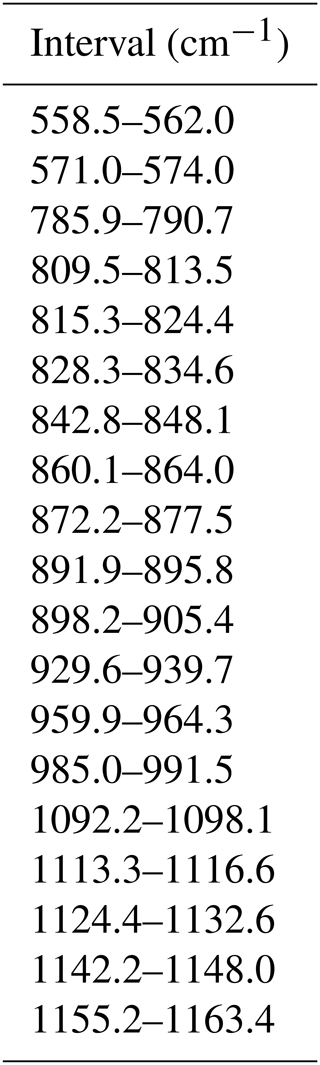The aim of the iterations is to minimize the cost function ξ2(x).

$\begin{array}{}\text{(B4)}& \begin{array}{rl}{\mathit{\xi }}^{\mathrm{2}}\left({\mathbit{x}}_{n}\right)& ={\left[\mathbit{y}-F\left({\mathbit{x}}_{n}\right)\right]}^{T}{\mathbf{S}}_{y}^{-\mathrm{1}}\left[\mathbit{y}-F\left({\mathbit{x}}_{n}\right)\right]\\ & +{\left[{\mathbit{x}}_{\mathrm{a}}-{\mathbit{x}}_{n}\right]}^{T}{\mathbf{S}}_{\mathrm{a}}^{-\mathrm{1}}\left[{\mathbit{x}}_{\mathrm{a}}-{\mathbit{x}}_{n}\right]\end{array}\end{array}$

Convergence is reached if the change in the cost function is below a given threshold, here set to 0.1 %:

$\begin{array}{}\text{(B5)}& \frac{{\mathit{\xi }}^{\mathrm{2}}\left({x}_{n+\mathrm{1}}\right)-{\mathit{\xi }}^{\mathrm{2}}\left({x}_{n}\right)}{{\mathit{\xi }}^{\mathrm{2}}\left({x}_{n+\mathrm{1}}\right)}<\mathrm{0.001}.\end{array}$

However, convergence in the sense of the cost function does not necessarily mean that the fitted and measured spectra match. For example, the step size parameter of the Levenberg–Marquardt method could be so large that the cost function changes little. Then the convergence criterion is fulfilled, but the fit does not agree with the measurement. To identify these cases, a reduced-χ2 test is performed. This test is used to calculate the distance between calculated and measured radiance, taking into account the variance of the spectrum σ2. It is defined as

$\begin{array}{}\text{(B6)}& {\mathit{\chi }}_{\mathrm{reduced}}^{\mathrm{2}}=\frac{\mathrm{1}}{\text{DOF}}\cdot \sum _{m=\mathrm{1}}^{N}\frac{y\left({\overline{\mathit{\nu }}}_{\mathrm{m}}\right)-F\left(\mathbf{x},{\overline{\mathit{\nu }}}_{\mathrm{m}}\right)}{{\mathit{\sigma }}^{\mathrm{2}}},\end{array}$

with DOF equal to the number of data points minus the number of parameters. The microwindow is denoted as ${\overline{\mathit{\nu }}}_{\mathrm{m}}$. As empirical values, we assume that all retrievals with ${\mathit{\xi }}_{\mathrm{reduced}}^{\mathrm{2}}<\mathrm{1.0}$ converged correctly. Results with ${\mathit{\tau }}_{\mathrm{liq}}+{\mathit{\tau }}_{\mathrm{ice}}>\mathrm{6}$ are excluded.

As we do not necessarily have prior information about the optical depths and effective radii, we decided to set the covariance of the a priori to large values. This ensures that the chosen a priori does not constrain the retrieval too strongly. Initial values and the a priori are set to equal values: ${\mathbit{x}}_{\mathrm{a}}=\left(\mathrm{0.25},\mathrm{0.25},\mathrm{log}\left(\mathrm{5.0}\right),\mathrm{log}\left(\mathrm{20.0}\right)\right)$. The logarithm was chosen so that all entries of xa have similar sizes. The variance–covariance matrix of the a priori is set to

$\begin{array}{}\text{(B7)}& {\mathbf{S}}_{\mathrm{a}}^{-\mathrm{1}}=\left(\begin{array}{cccc}\mathrm{0.04}& \mathrm{0}& \mathrm{0}& \mathrm{0}\\ \mathrm{0}& \mathrm{0.04}& \mathrm{0}& \mathrm{0}\\ \mathrm{0}& \mathrm{0}& \mathrm{0.047}& \mathrm{0}\\ \mathrm{0}& \mathrm{0}& \mathrm{0}& \mathrm{0.047}\end{array}\right).\end{array}$

The values in xa and ${\mathbf{S}}_{\mathrm{a}}^{-\mathrm{1}}$ are chosen empirically. Since initially no information about the cloud parameters is available, xa and ${\mathbf{S}}_{\mathrm{a}}^{-\mathrm{1}}$ should not restrict the retrieval too much. Therefore, the variances in ${\mathbf{S}}_{\mathrm{a}}^{-\mathrm{1}}$ are set to large values.

Variances in ${\mathbf{S}}_{y}^{-\mathrm{1}}$ are calculated from the spectral region between 1925 and 2000 cm−1, where no signal from the atmosphere is expected. The variance–covariance matrix is assumed to be diagonal: Sy=σ2I. It is assumed to be the variance of the scene. To retrieve cloud parameters, only radiance from spectral intervals given in Table B1 is used. The variances of Sy propagated into the covariance matrix Sr of the result by applying a transfer matrix T. In each step T is calculated taking into account the current step size parameter μ by

with 0 as the zero matrix and I as the identity matrix. Mi is the inverse of the term in the parentheses on the left side of Eq. (B3), and ${\mathbf{G}}_{i}={\mathbf{M}}_{i}{\mathbf{K}}_{i}^{T}{\mathbf{S}}_{y}^{-\mathrm{1}}$. Diagonal elements of Sr are the variances of the final cloud parameters.

Appendix C: Retrieval performance on simulated spectra

A set of simulated test cases containing spectral radiances of artificial clouds with known cloud parameters, created by , will be used to test the ability of TCWret to retrieve τliq, τice, rliq and rice. Additionally, the derived quantities LWP and IWP are discussed. This dataset contains several representative cases of Arctic clouds. Clouds are set to be vertically homogeneous, topped by a layer of liquid water or with thin boundaries. Ice crystal shapes are mostly set to be spheres, but some cases were calculated with hollow columns, solid columns, bullet rosettes or plates. All spectra are convoluted with a sinc function to the resolution of the IFS 55 Equinox (0.3 cm−1) and perturbed by a Gaussian-distributed noise of $\mathrm{1}\phantom{\rule{0.125em}{0ex}}\mathrm{mW}\phantom{\rule{0.125em}{0ex}}\left({\mathrm{m}}^{\mathrm{2}}\phantom{\rule{0.125em}{0ex}}\mathrm{sr}\phantom{\rule{0.125em}{0ex}}{\mathrm{cm}}^{-\mathrm{1}}{\right)}^{-\mathrm{1}}$: we modified the spectral radiance at each wavenumber by drawing a random number from a normal distribution with the true spectral radiance as the mean of the distribution and $\mathrm{1}\phantom{\rule{0.125em}{0ex}}\mathrm{mW}\phantom{\rule{0.125em}{0ex}}\left({\mathrm{m}}^{\mathrm{2}}\phantom{\rule{0.125em}{0ex}}\mathrm{sr}\phantom{\rule{0.125em}{0ex}}{\mathrm{cm}}^{-\mathrm{1}}{\right)}^{-\mathrm{1}}$ as its standard deviation. This value has been chosen because it is near the observed standard deviation of the real spectra from the measurement campaign of $\mathrm{0.82}\phantom{\rule{0.125em}{0ex}}\mathrm{mW}\phantom{\rule{0.125em}{0ex}}\left({\mathrm{m}}^{\mathrm{2}}\phantom{\rule{0.125em}{0ex}}\mathrm{sr}\phantom{\rule{0.125em}{0ex}}{\mathrm{cm}}^{-\mathrm{1}}{\right)}^{-\mathrm{1}}$. Ice crystals are chosen to be spheres; thus only the test cases which are calculated with spherical ice crystals are used here. The influence of the chosen ice particle form will be addressed later.

Table 3 gives the correlation coefficients, mean biases and standard deviations between the retrieved cloud parameters of the test cases and the true cloud parameters. Additionally, the standard deviations calculated via the variance–covariance matrix are given. TCWret is able to determine optical depths and effective radii of the simulated spectra.

Of all direct retrieval products, the optical depths τliq and τice have the highest agreement with the true cloud parameters. For the liquid phase, the difference from the true optical depths is $\left(-\mathrm{0.1}±\mathrm{0.5}\right)$. For the optical depth of the ice phase, the difference is larger with (0.2±0.6). Since τliq and τice include both optical depths and phase, the optical depth of the condensed water ${\mathit{\tau }}_{\mathrm{cw}}={\mathit{\tau }}_{\mathrm{liq}}+{\mathit{\tau }}_{\mathrm{ice}}$ as well as the fraction of ice in the optical depth ${f}_{\mathrm{ice}}={\mathit{\tau }}_{\mathrm{ice}}\cdot {\mathit{\tau }}_{\mathrm{cw}}^{-\mathrm{1}}$ are calculated. Here it becomes clear that the optical depth can be determined accurately ($|r|=\mathrm{0.99}$, mean bias and RMSE (0.1±0.2)). It then also follows that the deviations of τliq and τice come from the phase determination. The deviation for the phase is (0.1±0.3) with a correlation coefficient of $|r|=\mathrm{0.70}$.

When considering the effective radii, only results of rliq were used where fice is less than 0.9. For rice only results with fice>0.1 are considered. The mean difference in the retrieval from the true parameters and the root-mean-square error are $\left(-\mathrm{2.4}±\mathrm{4.1}\right)$ for rliq and (3.0±10.0) for rice.

To estimate the influence of the a priori on the calculated result, the averaging kernel matrix is used. The mean averaging kernel matrix over all retrievals is

$\begin{array}{}\text{(C1)}& \mathbf{A}=\left(\begin{array}{cccc}\mathrm{0.87}& \mathrm{0.09}& -\mathrm{0.15}& -\mathrm{0.09}\\ \mathrm{0.11}& \mathrm{0.90}& \mathrm{0.19}& \mathrm{0.03}\\ -\mathrm{0.04}& \mathrm{0.07}& \mathrm{0.50}& \mathrm{0.05}\\ -\mathrm{0.16}& \mathrm{0.05}& \mathrm{0.03}& \mathrm{0.42}\end{array}\right).\end{array}$

From Eq. (8) it can be seen that the diagonal elements show for each parameter how strongly the retrieved parameter is influenced by the a priori. Whereas the diagonal elements of the optical depths are near 1, indicating independence from the a priori, results for rliq and rice show a larger influence from the a priori. From the trace of the averaging kernels follow 2.69 degrees of freedom of the signal.

The water paths are calculated from the optical depths and effective radii; therefore both quantities are influenced by the phase determination, as seen before in τliq,ice and rliq,ice. The difference from the test cases is $\left(-\mathrm{1.6}±\mathrm{6.3}\right)$ for the LWP and (1.9±10.0) for the IWP. However, the RMSE for the LWP is less than the minimum RMSE observed for the LWP from microwave radiometer of at least 15 g m−2 .

Standard deviations given by the variance–covariance matrix of the retrieval are shown in Table 3 and named ERR(OE). ERR(OE) is below RMSE for τliq,ice, rliq,ice, the LWP and the IWP. This might be due to uncertainties from the forward model – which are neglected here – propagated into the retrievals or due to the assumption of a diagonal variance matrix Sy. To compensate for these effects, the uncertainties from the posterior covariance matrix are scaled by RMSE $/$ ERR(OE) with the RMSE from Table 3 for the discussion in Sect. 5.

## C1 Mean bias and RMSE of effective radii

In the previous section, the results for rliq and rice were only considered for a certain range of fice. Thus, liquid drops were only included in the consideration if the ice content was not higher than 90 %. For ice crystals, the limit was at least 10 % ice content. In the following, these limits are shifted so that the results go in the direction of a single-phase retrieval for liquid water and ice.

Table C1 shows the results for liquid water. The entries at the top describe cases with a higher proportion of liquid water than the cases at the bottom, which allow a higher proportion of ice. They are cumulative, which means that each record also contains the data of the record above it. From the test cases it follows that the fewer ice crystals present, the lower the RMSE. Also, the absolute mean bias decreases with lower ice content up to an ice content between 10 % and 30 %. These results indicate that the presence of ice crystals lead to an underestimation of rliq by TCWret.

Table C2 show the results for ice crystals. Here we introduced fliq, which is defined as ${f}_{\mathrm{liq}}=\mathrm{1}-{f}_{\mathrm{ice}}$, to create a table consistent with Table C1. Here one can see that the RMSE of rice is almost independent of the water content. However, there is a dependence of the mean bias on water content. While removing clouds with very high water content leads to a decrease in absolute mean bias, the absolute value of mean bias increases for clouds with high ice content, so TCWret underestimates rice of the simulated spectra.

Appendix D: Influence of trace gas concentrations on the retrieval

In the LBLRTM, a standard atmosphere was used for gases except water vapor. Therefore, the concentration of CO2 is set to 330 ppm, although the real concentration in summer 2017 is about 410 ppm. To investigate the influence of an incorrect trace gas concentration, retrievals from the 11 June 2016 have been performed with both atmospheric concentrations of CO2. Differences are calculated for the cloud parameters τliq, τice, rliq and rice and shown in Fig. D1. For all parameters, correlation coefficients between $|r|=\mathrm{0.98}$ and $|r|=\mathrm{1.00}$ can be observed. The maximum mean bias is observed for rliq (0.1 µm), and the maximum RMSE is observed for rice (1.3 µm). From this it can be concluded that the influence of the trace gas concentration is negligible compared to the other uncertainties.Figure D1Histograms of differences for CO2 concentrations of 410 and 330 ppm for τliq, τice, rliq and rice.

Appendix E: Ice crystal shapes in the netCDF file

Table E1 refers to each key in the field ice_shape in the netCDF file and the corresponding ice crystal shape.

Author contributions

PR performed measurements during PS106 and PS107, implemented TCWret, and performed retrievals from infrared spectra. MP designed and built the measurement setup, performed measurements during PS106.1, measured the emissivity of the blackbody radiator and gave advice on the development of TCWret. CW performed measurements during PS106.2 and built the measurement setup. HG performed Cloudnet retrievals and gave advice on using the Cloudnet data. PMR gave advice on the application of the test cases. JN gave advice on the setup of the measurement and the development of TCWret. All authors contributed to manuscript revisions.

Competing interests

The contact author has declared that neither they nor their co-authors have any competing interests.

Disclaimer

Publisher's note: Copernicus Publications remains neutral with regard to jurisdictional claims in published maps and institutional affiliations.

Special issue statement

This article is part of the special issue “Arctic mixed-phase clouds as studied during the ACLOUD/PASCAL campaigns in the framework of (AC)3 (ACP/AMT/ESSD inter-journal SI)”. It is not associated with a conference.

Acknowledgements

We gratefully acknowledge funding from the Deutsche Forschungsgemeinschaft (DFG, German Research Foundation, TRR 172) – Projektnummer 268020496 – within the Transregional Collaborative Research Center – ArctiC Amplification: Climate Relevant Atmospheric and SurfaCe Processes, and Feedback Mechanisms (AC)3 – in subproject B06 and E02. We thank the Alfred Wegener Institute and RV Polarstern crew and captain for their support (AWI_PS106_00 and AWI_PS107_00). The computations were performed on the HPC cluster Aether at the University of Bremen, financed by DFG within the scope of the Excellence Initiative.

Financial support

This research has been supported by the Deutsche Forschungsgemeinschaft (grant no. 268020496).

Review statement

This paper was edited by Timo Vihma and reviewed by two anonymous referees.

References

Becker, E. and Notholt, J.: Intercomparison and validation of FTIR measurements with the Sun, the Moon and emission in the Arctic, J. Quant. Spectrosc. Ra., 65, 779–786, https://doi.org/10.1016/S0022-4073(99)00154-5, 2000. a

Becker, E., Notholt, J., and Herber, A.: Tropospheric aerosol measurements in the Arctic by FTIR-emission and star photometer extinction spectroscopy, Geophys. Res. Lett., 26, 1711–1714, https://doi.org/10.1029/1999GL900336, 1999. a

Ceccherini, S. and Ridolfi, M.: Technical Note: Variance-covariance matrix and averaging kernels for the Levenberg-Marquardt solution of the retrieval of atmospheric vertical profiles, Atmos. Chem. Phys., 10, 3131–3139, https://doi.org/10.5194/acp-10-3131-2010, 2010. a, b

Clough, S. A., Shephard, M. W., Mlawer, E. J., Delamere, J. S., Iacono, M. J., Cady-Pereira, K., Boukabara, S., and Brown, P. D.: Atmospheric radiative transfer modeling: a summary of the AER codes, J. Quant. Spectros. Ra., 91, 233–244, 2005. a, b

Cox, C. J., Walden, V. P., Rowe, P. M., and Shupe, M. D.: Humidity trends imply increased sensitivity to clouds in a warming Arctic, Nat. Commun., 6, 10117, https://doi.org/10.1038/ncomms10117, 2015. a

Cox, C. J., Rowe, P. M., Neshyba, S. P., and Walden, V. P.: A synthetic data set of high-spectral-resolution infrared spectra for the Arctic atmosphere, Earth Syst. Sci. Data, 8, 199–211, https://doi.org/10.5194/essd-8-199-2016, 2016. a, b, c

Downing, H. D. and Williams, D.: Optical constants of water in the infrared, J. Geophys. Res., 80, 1656–1661, https://doi.org/10.1029/JC080i012p01656, 1975. a

Frisch, S., Shupe, M., Djalalova, I., Feingold, G., and Poellot, M.: The Retrieval of Stratus Cloud Droplet Effective Radius with Cloud Radars, J. Atmos. Ocean. Tech., 19, 835–842, https://doi.org/10.1175/1520-0426(2002)019<0835:TROSCD>2.0.CO;2, 2002. a

Griesche, H., Seifert, P., Engelmann, R., Radenz, M., and Bühl, J.: OCEANET-ATMOSPHERE Microwave Radiometer HATPRO during PS106, PANGAEA [data set], https://doi.org/10.1594/PANGAEA.919359, 2020a. a

Griesche, H., Seifert, P., Engelmann, R., Radenz, M., and Bühl, J.: Cloudnet IWC during PS106, PANGAEA [data set], https://doi.org/10.1594/PANGAEA.919452, 2020b. a

Griesche, H., Seifert, P., Engelmann, R., Radenz, M., and Bühl, J.: Cloudnet ice particles effective radius during PS106, PANGAEA [data set], https://doi.org/10.1594/PANGAEA.919386, 2020c. a

Griesche, H., Seifert, P., Engelmann, R., Radenz, M., and Bühl, J.: Cloudnet LWC during PS106, PANGAEA [data set], https://doi.org/10.1594/PANGAEA.919383, 2020d. a

Griesche, H., Seifert, P., Engelmann, R., Radenz, M., and Bühl, J.: Cloudnet liquid droplet effective radius during PS106, PANGAEA [data set], https://doi.org/10.1594/PANGAEA.919399, 2020e. a

Griesche, H. J., Seifert, P., Ansmann, A., Baars, H., Barrientos Velasco, C., Bühl, J., Engelmann, R., Radenz, M., Zhenping, Y., and Macke, A.: Application of the shipborne remote sensing supersite OCEANET for profiling of Arctic aerosols and clouds during Polarstern cruise PS106, Atmos. Meas. Tech., 13, 5335–5358, https://doi.org/10.5194/amt-13-5335-2020, 2020f. a, b, c

Hersbach, H., Bell, B., Berrisford, P., Biavati, G., Horányi, A., Muñoz Sabater, J., Nicolas, J., Peubey, C., Radu, R., Rozum, I., Schepers, D., Simmons, A., Soci, C., Dee, D., and Thépaut, J.-N.: ERA5 hourly data on pressure levels from 1979 to present. Copernicus Climate Change Service (C3S) Climate Data Store (CDS) [data set], https://doi.org/10.24381/cds.bd0915c6, 2018. a

Hogan, R. J., Mittermaier, M. P., and Illingworth, A. J.: The Retrieval of Ice Water Content from Radar Reflectivity Factor and Temperature and Its Use in Evaluating a Mesoscale Model, J. Appl. Meteorol. Climatol., 45, 301–317, https://doi.org/10.1175/JAM2340.1, 2006. a

Illingworth, A. J., Hogan, R. J., O'Connor, E., Bouniol, D., Brooks, M. E., Delanoé, J., Donovan, D. P., Eastment, J. D., Gaussiat, N., Goddard, J. W. F., Haeffelin, M., Baltink, H. K., Krasnov, O. A., Pelon, J., Piriou, J.-M., Protat, A., Russchenberg, H. W. J., Seifert, A., Tompkins, A. M., van Zadelhoff, G.-J., Vinit, F., Willén, U., Wilson, D. R., and Wrench, C. L.: Cloudnet, B. Am. Meteorol. Soc., 88, 883–898, https://doi.org/10.1175/BAMS-88-6-883, 2007. a

Knuteson, R. O., Revercomb, H. E., Best, F. A., Ciganovich, N. C., Dedecker, R. G., Dirckx, T. P., Ellington, S. C., Feltz, W. F., Garcia, R. K., Howell, H. B., Smith, W. L., Short, J. F., and Tobin, D. C.: Atmospheric Emitted Radiance Interferometer. Part II: Instrument Performance, J. Atmos. Ocean. Tech., 21, 1777–1789, https://doi.org/10.1175/JTECH-1663.1, 2004a. a

Knuteson, R. O., Revercomb, H. E., Best, F. A., Ciganovich, N. C., Dedecker, R. G., Dirkx, T. P., Ellington, S. C., Feltz, W. F., Garcia, R. K., Howell, H. B., Smith, W. L., Short, J. F., and Tobin, D. C.: Atmospheric Emitted Radiance Interferometer. Part I: Instrument Design, J. Atmos. Ocean. Tech., 21, 1763–1776, https://doi.org/10.1175/JTECH-1662.1, 2004b. a

Löhnert, U. and Crewell, S.: Accuracy of cloud liquid water path from ground-based microwave radiometry 1. Dependency on cloud model statistics, Radio Sci., 38, 8041, https://doi.org/10.1029/2002RS002654, 2003. a, b, c

Macke, A. and Flores, H.: The Expeditions PS106/1 and 2 of the Research Vessel POLARSTERN to the Arctic Ocean in 2017, TIB, https://doi.org/10.2312/BzPM_0719_2018, 2018. a

Rathke, C. and Fischer, J.: Retrieval of Cloud Microphysical Properties from Thermal Infrared Observations by a Fast Iterative Radiance Fitting Method, J. Atmos. Ocean. Tech., 17, 1509–1524, https://doi.org/10.1175/1520-0426(2000)017<1509:ROCMPF>2.0.CO;2, 2000. a, b

Rathke, C., Fischer, J., Neshyba, S., and Shupe, M.: Improving IR cloud phase determination with 20 microns spectral observations, Geophys. Res. Lett., 29, 51–54, https://doi.org/10.1029/2001GL014594, 2002. a

Revercomb, H. E., Buijs, H., Howell, H. B., Laporte, D. D., Smith, W. L., and Sromovsky, L. A.: Radiometric calibration of IR Fourier transform spectrometers: solution to a problem with the High-Resolution Interferometer Sounder, Appl. Optics, 27, 3210–3218, https://doi.org/10.1364/AO.27.003210, 1988. a, b

Richter, P.: Total Cloud Water retrieval, Zenodo [code], https://doi.org/10.5281/zenodo.4621127, 2021a. a

Richter, P.: RichterIUP/run_LBLRTM: run_LBLRTM (1.0), Zenodo [code], https://doi.org/10.5281/zenodo.4618142, 2021b. a

Richter, P.: RichterIUP/run_LBLDIS: run_LBLDIS (1.0), Zenodo [code], https://doi.org/10.5281/zenodo.4618106, 2021c. a

Richter, P.: RichterIUP/evaluation_tcwret: evaluation_tcwret (1.0), Zenodo [code], https://doi.org/10.5281/zenodo.6647314, 2022. a

Richter, P., Palm, M., Weinzierl, C., and Notholt, J.: Microphysical Cloud Parameters and water paths retrieved from EM-FTIR spectra, measured during PS106 and PS107 in Arctic summer 2017, PANGAEA [data set], https://doi.org/10.1594/PANGAEA.933829, 2021. a, b

Rodgers, C.: Inverse Methods for Atmospheric Sounding: Theory and Practice, World Scientific, https://doi.org/10.1142/3171, 2000. a, b, c

Rowe, P. M., Neshyba, S., and Walden, V. P.: Radiative consequences of low-temperature infrared refractive indices for supercooled water clouds, Atmos. Chem. Phys., 13, 11925–11933, https://doi.org/10.5194/acp-13-11925-2013, 2013. a

Rowe, P. M., Cox, C. J., Neshyba, S., and Walden, V. P.: Toward autonomous surface-based infrared remote sensing of polar clouds: retrievals of cloud optical and microphysical properties, Atmos. Meas. Tech., 12, 5071–5086, https://doi.org/10.5194/amt-12-5071-2019, 2019. a

Schewe, I.: The Expedition PS107 of the Research Vessel POLARSTERN to the Fram Strait and the AWI-HAUSGARTEN in 2017, TIB, https://doi.org/10.2312/BzPM_0717_2018, 2018. a

Schmithüsen, H.: Ceilometer CL51 raw data measured during POLARSTERN cruise PS106.1, links to files, PANGAEA [data set], https://doi.org/10.1594/PANGAEA.883320, 2017a. a, b

Schmithüsen, H.: Ceilometer CL51 raw data measured during POLARSTERN cruise PS106.2, links to files, PANGAEA [data set], https://doi.org/10.1594/PANGAEA.883322, 2017b. a, b

Schmithüsen, H.: Ceilometer CL51 raw data measured during POLARSTERN cruise PS107, links to files, PANGAEA [data set], https://doi.org/10.1594/PANGAEA.883323, 2017c. a, b

Schmithüsen, H.: Upper air soundings during POLARSTERN cruise PS106.1 (ARK-XXXI/1.1), PANGAEA [data set], https://doi.org/10.1594/PANGAEA.882736, 2017d. a, b

Schmithüsen, H.: Upper air soundings during POLARSTERN cruise PS106.2 (ARK-XXXI/1.2), PANGAEA [data set], https://doi.org/10.1594/PANGAEA.882843, 2017e. a, b

Schmithüsen, H.: Upper air soundings during POLARSTERN cruise PS107 (ARK-XXXI/2), PANGAEA [data set], https://doi.org/10.1594/PANGAEA.882889, 2017f. a, b

Shupe, M. and Intrieri, J.: Cloud Radiative Forcing of the Arctic Surface: The Influence of Cloud Properties, Surface Albedo, and Solar Zenith Angle, J. Climate, 17, 616–628, https://doi.org/10.1175/1520-0442(2004)017<0616:CRFOTA>2.0.CO;2, 2004. a

Stamnes, K., Tsay, S. C., Wiscombe, W., and Jayaweera, K.: Numerically stable algorithm for discrete-ordinate-method radiative transfer in multiple scattering and emitting layered media, Appl. Optics, 27, 2502–2509, 1988. a, b

Turner, D. D.: Arctic Mixed-Phase Cloud Properties from AERI Lidar Observations: Algorithm and Results from SHEBA, Americal Meteorological Society, 427–444, 2005. a, b, c, d, e, f

Turner, D. D.: Additional Information for LBLDIS, https://web.archive.org/web/20170508194542/http://www.nssl.noaa.gov/users/dturner/public_html/lbldis/index.html (last access: 8 May 2017), 2014. a

Turner, D. D., Vogelmann, A. M., Baum, B. A., Revercomb, H. E., and P., Y.: Cloud Phase Determination Using Ground-Based AERI Observations at SHEBA, Americal Meteorological Society, 701–715, 2003. a

Turner, D. D., Vogelmann, A. M., Austin, R. T., Barnard, J. C., Cady-Pereira, K., Chiu, J. C., Clough, S. A., Flynn, C., Khaiyer, M. M., Liljegren, J., Johnson, K., Lin, B., Long, C., Marshak, A., Matrosov, S. Y., McFarlane, S. A., Miller, M., Min, Q., Minnis, P., O'Hirok, W., Wang, Z., and Wiscombe, W.: Thin Liquid Water Clouds – Their Importance and Our Challenge, Americal Meteorological Society, 177–190, https://doi.org/10.1175/BAMS-88-2-177, 2007. a, b

Warren, S. G.: Optical constants of ice from the ultraviolet to the microwave, Appl. Optics, 23, 1206–1225, https://doi.org/10.1364/AO.23.001206, 1984. a

Wendisch, M., Macke, A., Ehrlich, A., Lüpkes, C., Mech, M., Chechin, D., Dethloff, K., Velasco, C. B., Bozem, H., Brückner, M., Clemen, H.-C., Crewell, S., Donth, T., Dupuy, R., Ebell, K., Egerer, U., Engelmann, R., Engler, C., Eppers, O., Gehrmann, M., Gong, X., Gottschalk, M., Gourbeyre, C., Griesche, H., Hartmann, J., Hartmann, M., Heinold, B., Herber, A., Herrmann, H., Heygster, G., Hoor, P., Jafariserajehlou, S., Jäkel, E., Järvinen, E., Jourdan, O., Kästner, U., Kecorius, S., Knudsen, E. M., Köllner, F., Kretzschmar, J., Lelli, L., Leroy, D., Maturilli, M., Mei, L., Mertes, S., Mioche, G., Neuber, R., Nicolaus, M., Nomokonova, T., Notholt, J., Palm, M., van Pinxteren, M., Quaas, J., Richter, P., Ruiz-Donoso, E., Schäfer, M., Schmieder, K., Schnaiter, M., Schneider, J., Schwarzenböck, A., Seifert, P., Shupe, M. D., Siebert, H., Spreen, G., Stapf, J., Stratmann, F., Vogl, T., Welti, A., Wex, H., Wiedensohler, A., Zanatta, M., and Zeppenfeld, S.: The Arctic Cloud Puzzle: Using ACLOUD/PASCAL Multiplatform Observations to Unravel the Role of Clouds and Aerosol Particles in Arctic Amplification, B. Am. Meteorol. Soc., 100, 841–871, https://doi.org/10.1175/BAMS-D-18-0072.1, 2019.  a, b

Yang, P., Gao, B.-C., Baum, B. A., Hu, Y. X., Wiscombe, W. J., Tsay, S.-C., Winker, D. M., and Nasiri, S. L.: Radiative properties of cirrus clouds in the infrared (8–13 µm) spectral region, J. Quant. Spectrosc. Ra., 70, 473–504, https://doi.org/10.1016/S0022-4073(01)00024-3, 2001. a

Yang, P., Zhang, L., Hong, G., Nasiri, S. L., Baum, B. A., Huang, H., King, M. D., and Platnick, S.: Differences Between Collection 4 and 5 MODIS Ice Cloud Optical/Microphysical Products and Their Impact on Radiative Forcing Simulations, IEEE T. Geosci. Remote, 45, 2886–2899, https://doi.org/10.1109/TGRS.2007.898276, 2007. a

Zasetsky, A. Y., Khalizov, A. F., Earle, M. E., and Sloan, J. J.: Frequency Dependend Complex Refractive Indices of Supercooled Liquid Water and Ice Determined from Aerosol Extinction Spectra, J. Phys. Chem. A, 109, 2760–2764, https://doi.org/10.1021/jp044823c, 2005. a